xLowest common denominatorEncyclopedia
In mathematics
Mathematics
Mathematics is the study of quantity, space, structure, and change. Mathematicians seek out patterns and formulate new conjectures. Mathematicians resolve the truth or falsity of conjectures by mathematical proofs, which are arguments sufficient to convince other mathematicians of their validity...

, the lowest common denominator or least common denominator (abbreviated LCD) is the least common multiple
Least common multiple
In arithmetic and number theory, the least common multiple of two integers a and b, usually denoted by LCM, is the smallest positive integer that is a multiple of both a and b...

of the denominators of a set of vulgar fractions. It is the smallest positive integer
Integer
The integers are formed by the natural numbers together with the negatives of the non-zero natural numbers .They are known as Positive and Negative Integers respectively...

that is a multiple of the denominators.

The term is also used in popular culture with a different though related meaning.

## Examples

The LCD of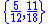is 36 because the least common multiple
Least common multiple
In arithmetic and number theory, the least common multiple of two integers a and b, usually denoted by LCM, is the smallest positive integer that is a multiple of both a and b...

of 12 and 18 is 36. Likewise the LCD of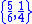is 12. Using the LCD (or any multiple of it, such as the product of the denominators) as a denominator enables addition
Addition is a mathematical operation that represents combining collections of objects together into a larger collection. It is signified by the plus sign . For example, in the picture on the right, there are 3 + 2 apples—meaning three apples and two other apples—which is the same as five apples....

, subtraction
Subtraction
In arithmetic, subtraction is one of the four basic binary operations; it is the inverse of addition, meaning that if we start with any number and add any number and then subtract the same number we added, we return to the number we started with...

or comparison of fractions: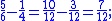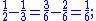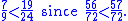The lowest common denominator of two vulgar fractions can be found by calculating the least common multiple of their denominators.

## Middle school instruction

Some K–1 math standards such as the latest revision of the NCTM math standards and reform mathematics
Reform mathematics
Reform mathematics is an approach to mathematics education, particularly in North America. It is based on principles explained in 1989 by the National Council of Teachers of Mathematics . The NCTM document, Curriculum and Evaluation Standards for School Mathematics, attempted to set forth a vision...

textbooks created since the 1990s de-emphasize or omit coverage of the LCD entirely in favor of finding any common, but not necessarily the lowest common denominator, or by using less powerful methods such as fraction strips or "benchmark" fractions. The "cross-multiply" method of comparing fractions effectively creates a common denominator by multiplying both denominators together.

Algorithm finds lowest common denominator.

Lowest common denominator for 2/9 + 1/4 + 1/6:

The algorithm uses similar division boxes going downward.

Start with 2 and see if it divides exactly into any of the three denominators.
Then go to 3, then 4, then 5, and so on.

|_9_4_6_

2|_9_4_6_ 2 doesn't go into 9 exactly. 2 goes into 4 two times, and into 6 three times.

2|_9_2_3_ 2 goes into 2 one time.

3|_9_1_3_ 3 is the next divisor. 3 goes into 9 three times, and into 3 one time.

3|_3_1_1_ 3 goes into 3 one time.

|_1_1_1_

The process is to keep dividing the denominators until they reduce to 1.
Then ignore the 1's and use the column of divisors as factors which produce the L.C.D.

2 x 2 x 3 x 3 = 36 = L.C.D.

## Non-mathematical usage

'Lowest common denominator' is often used as a figure of speech meaning the most basic, least sophisticated level of taste
Taste (sociology)
Taste as an aesthetic, sociological, economic and anthropological concept refers to a cultural patterns of choice and preference. While taste is often understood as a biological concept, it can also be reasonably studied as a social or cultural phenomenon. Taste is about drawing distinctions...

, sensibility, or opinion among a group of people.

This popular sense of the term is not faithful to mathematical usage. Because the figure of speech conveys the sense of degrading by eliminating anything not common to all (but not degrading further), a better mathematical metaphor
Metaphor
A metaphor is a literary figure of speech that uses an image, story or tangible thing to represent a less tangible thing or some intangible quality or idea; e.g., "Her eyes were glistening jewels." Metaphor may also be used for any rhetorical figures of speech that achieve their effects via...

for the colloquial usage's connotation
Connotation
A connotation is a commonly understood subjective cultural or emotional association that some word or phrase carries, in addition to the word's or phrase's explicit or literal meaning, which is its denotation....

is 'greatest common divisor
Greatest common divisor
In mathematics, the greatest common divisor , also known as the greatest common factor , or highest common factor , of two or more non-zero integers, is the largest positive integer that divides the numbers without a remainder.For example, the GCD of 8 and 12 is 4.This notion can be extended to...

.' However, it seems that the word greatest strikes non-mathematicians' intuition as suggesting the lofty, rather than the base.